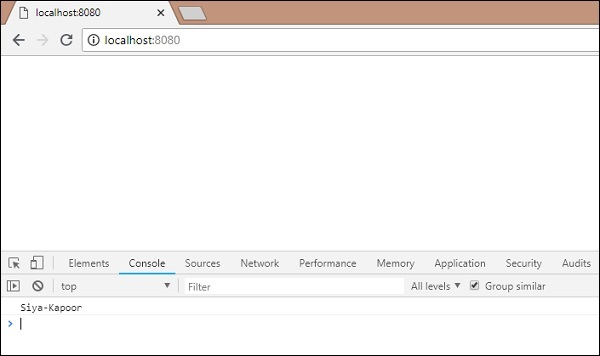# BabelJS - Working with Babel and Webpack

Webpack is a module bundler which packs all modules with dependencies – js, styles, images, etc. into static assets .js, .css, .jpg , .png, etc. Webpack comes with presets which help for compilation into the required form. For example, react preset that helps to get the final output in react form, es2015 or env preset that helps to compile the code in ES5 or 6 or 7, etc. We have used babel 6 in the project setup. In case you want to switch to babel7, install the required packages of babel using @babel/babel-package-name.

Here, we will discuss project setup using babel and webpack. Create a folder called and open the same in visual studio IDE.

To create the project setup, run npm initbabelwebpack as follows −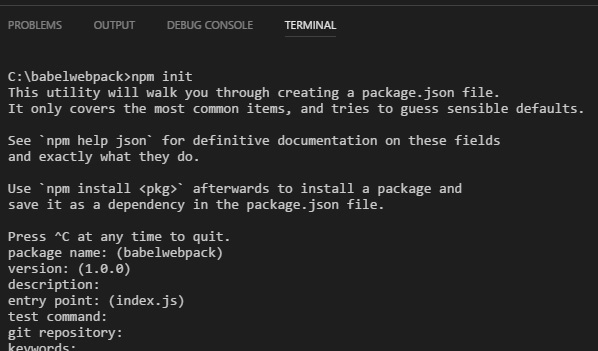Here is the package.json created after npm init −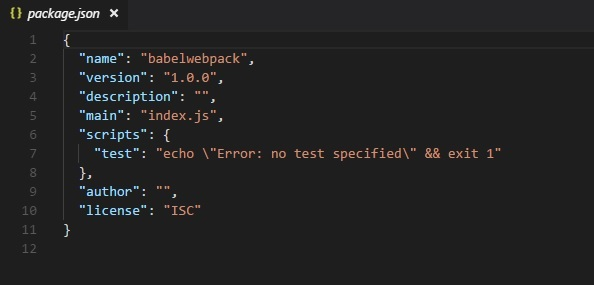Now, we will install the necessary packages we need to work with babel and webpack.

```npm install --save-dev webpack
npm install --save-dev webpack-dev-server
npm install --save-dev babel-core
npm install --save-dev babel-preset-env
```

Here is the Package.json after installation −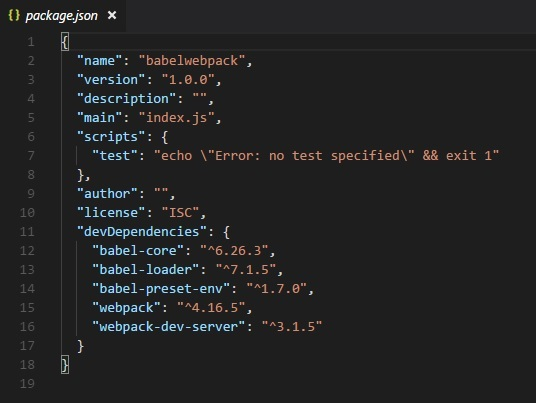Now, we will create a webpack.config.js file, which will have all the details to bundle the js files. These files will be compiled it into es5 using babel.

To run webpack using server, we use webpack-server. Following are the details added to it −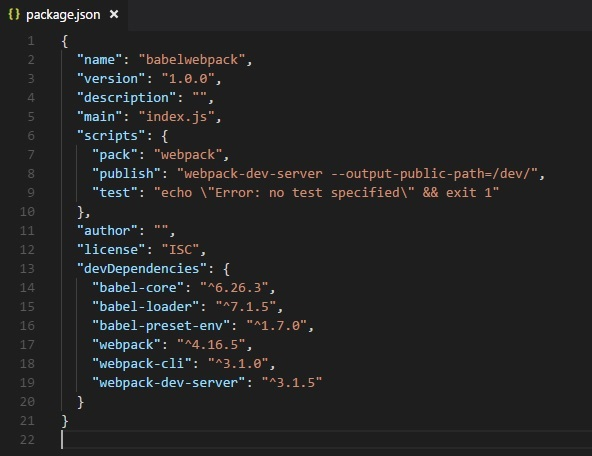We have added the publish command which will start the webpack-dev-server and will update the path where the final files are stored. Right now the path that we are going to use to update the final files is the /dev folder.

To use webpack, we need to run the following command −

```npm run publish
```

First we need to create the webpack.config.js files. These will have the configuration details for webpack to work.

The details in the file are as follows −

```var path = require('path');

module.exports = {
entry: {
app: './src/main.js'
},
output: {
path: path.resolve(__dirname, 'dev'),
filename: 'main_bundle.js'
},
mode:'development',
module: {
rules: [
{
test: /\.js\$/,
include: path.resolve(__dirname, 'src'),
query: {
presets: ['env']
}
}
]
}
};
```

The structure of the file is as shown above. It starts with theh path, which gives the current path details.

```var path = require('path'); //gives the current path
```

Next is the module.exports object, which has properties entry, output and module. The entry is the start point. Here, we need to give the main js files that has to be compiled.

```entry: {
app: './src/main.js'
},
```

path.resolve(_dirname, ‘src/main.js’) -- will look for the src folder in the directory and main.js in that folder.

### Output

```output: {
path: path.resolve(__dirname, 'dev'),
filename: 'main_bundle.js'
},
```

Output is an object with path and filename details. Path will hold the folder in which the compiled file will be kept and filename will tell the name of final file to be used in your .html file.

### module

```module: {
rules: [
{
test: /\.js\$/,
include: path.resolve(__dirname, 'src'),
query: {
presets: ['env']
}
}
]
}
```
• Module is an object with details of the rules. It has the following properties −

• test
• include
• query
• Test will hold details of all the js files ending with .js. It has the pattern, which will look for .js at the end in the entry point given.

• Include instructs the folder in use on the files to be looked at.

• Query has property presets, which is an array with value env – es5 or es6 or es7.

Create folder src and main.js in it; write your js code in ES6. Later, run the command to see it getting compiled to es5 using webpack and babel.

src/main.js

```let add = (a,b) => {
return a+b;
};
console.log(c);
```

Run the command −

```npm run pack
```

The compiled file looks as follows −

dev/main_bundle.js

```!function(e) {
var t = {};
function r(n) {
if(t[n])return t[n].exports;var o = t[n] = {i:n,l:!1,exports:{}};
return e[n].call(o.exports,o,o.exports,r),o.l=!0,o.exports
}
r.m = e,r.c = t,r.d = function(e,t,n) {
r.o(e,t)||Object.defineProperty(e,t,{enumerable:!0,get:n})
},
r.r = function(e) {
"undefined"!=typeof Symbol&&Symbol.toStringTag&&Object.defineProperty(e,Symbol.toStringTag,{value:"Module"}),Object.defineProperty(e,"__esModule",{value:!0})
},
r.t = function(e,t) {
if(1&t&&(e = r(e)),8&t)return e;
if(4&t&&"object"==typeof e&&e&&e.__esModule)return e;
var n = Object.create(null);
if(r.r(n),Object.defineProperty(n,"default",{enumerable:!0,value:e}),2&t&&"string"!=typeof e)for(var o in e)r.d(n,o,function(t) {return e[t]}.bind(null,o));
return n
},
r.n = function(e) {
var t = e&&e.__esModule?function() {return e.default}:function() {return e};
return r.d(t,"a",t),t
},
r.o = function(e,t) {return Object.prototype.hasOwnProperty.call(e,t)},
r.p = "",r(r.s = 0)
}([function(e,t,r) {"use strict";var n = function(e,t) {return e+t}(10,20);console.log(n)}]);
!function(e) {
var t = {};
function r(n) {
if(t[n])return t[n].exports;
var o = t[n] = {i:n,l:!1,exports:{}};
return e[n].call(o.exports,o,o.exports,r),o.l=!0,o.exports
}
r.m = e,r.c = t,r.d = function(e,t,n) {
r.o(e,t)||Object.defineProperty(e,t,{enumerable:!0,get:n})
},
r.r = function(e) {
"undefined"!=typeof Symbol&&Symbol.toStringTag&&Object.defineProperty(e,Symbol.toStringTag,{value:"Module"}),Object.defineProperty(e,"__esModule",{value:!0})
},
r.t = function(e,t) {
if(1&t&&(e=r(e)),
8&t)return e;
if(4&t&&"object"==typeof e&&e&&e.__esModule)return e;
var n = Object.create(null);
if(
r.r(n),
Object.defineProperty(n,"default",{enumerable:!0,value:e}),
2&t&&"string"!=typeof e
)
for(var o in e)r.d(n,o,function(t) {return e[t]}.bind(null,o));
return n
},
r.n = function(e) {
var t = e&&e.__esModule?function() {return e.default}:function() {return e};
return r.d(t,"a",t),t
},
r.o = function(e,t) {
return Object.prototype.hasOwnProperty.call(e,t)
},
r.p = "",r(r.s = 0)
}([function(e,t,r) {
"use strict";
var n = function(e,t) {return e+t}(10,20);
console.log(n)
}]);
```

The code is compiled as shown above. Webpack adds some code which is required internally and the code from main.js is seen at the end. We have consoled the value as shown above.

Add the final js file in .html file as follows −

```<html>
<body>
<script type="text/javascript" src="dev/main_bundle.js"></script>
</body>
</html>
```

Run the command −

```npm run publish
```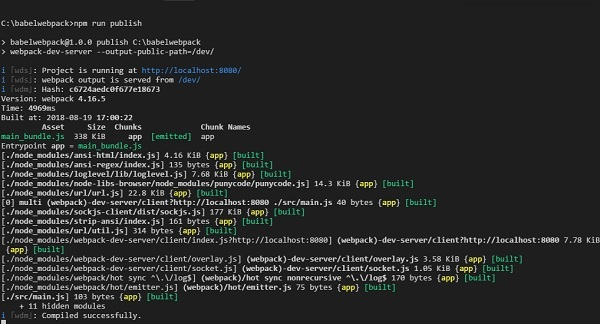To check the output, we can open the file in −

http://localhost:8080/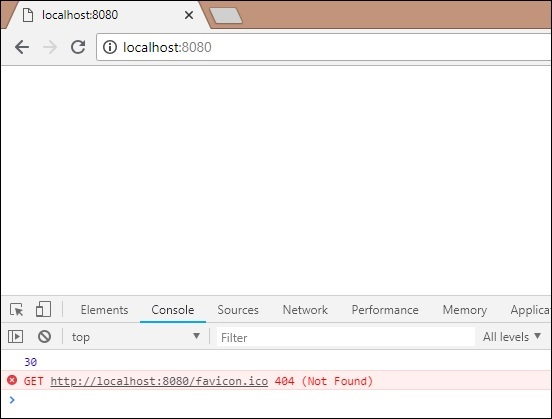We get the console value as shown above. Now let us try to compile to a single file using webpack and babel.

We will use webpack to bundle multiple js files into a single file. Babel will be used to compile the es6 code to es5.

Now, we have 2 js files in the src/ folder - main.js and Person.js as follows −

person.js

```export class Person {
this.fname = fname;
this.lname = lname;
this.age = age;
}

get fullname() {
return this.fname +"-"+this.lname;
}
}
```

We have used export to use the details of the Person class.

main.js

```import {Person} from './person'
var a = new Person("Siya", "Kapoor", "15", "Mumbai");
var persondet = a.fullname;
console.log(persondet);
```

In main.js, we have imported Person from the file path.

Note − We do not have to include person.js but just the name of the file. We have created an object of Person class and consoled the details as shown above.

Webpack will combine person.js and main.js and update in dev/main_bundle.js as one file. Run the command npm run publish to check the output in the browser −